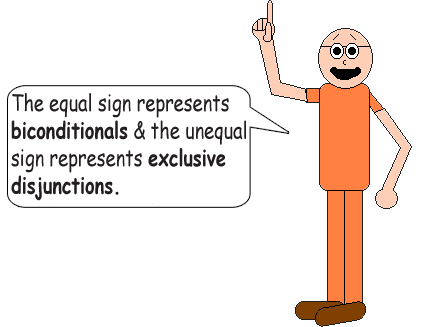# Single Simple Statements Can Create Tautologies!

## This math trick is about logic. A single simple statement can be used to create tautologies! (Or contradictions if you use the "NOT" function) The 1st truth table below uses biconditionals & exclusive disjunctions.

 P ~P P = P ~(P = P) P ≠ P ~(P ≠ P) (P = P) = ~(P ≠ P) ~(P = P) = (P ≠ P) TRUE FALSE TRUE FALSE FALSE TRUE TRUE TRUE FALSE TRUE TRUE FALSE FALSE TRUE TRUE TRUEThe tilde (~) represents the negation function in logic. When you use the word not in a sentence, you are using the negation function. It flips the truth value of a statement.

## Using 1 simple statement also makes tautologies with the conditional(If...then...) function! But not with conjunctions or inclusive disjunctions. The "neither...nor..." function can't make tautologies or contradictions with 1 simple statement, either.

 P ~P P & P P or P P→P P nor P TRUE FALSE TRUE TRUE TRUE FALSE FALSE TRUE FALSE FALSE TRUE TRUE